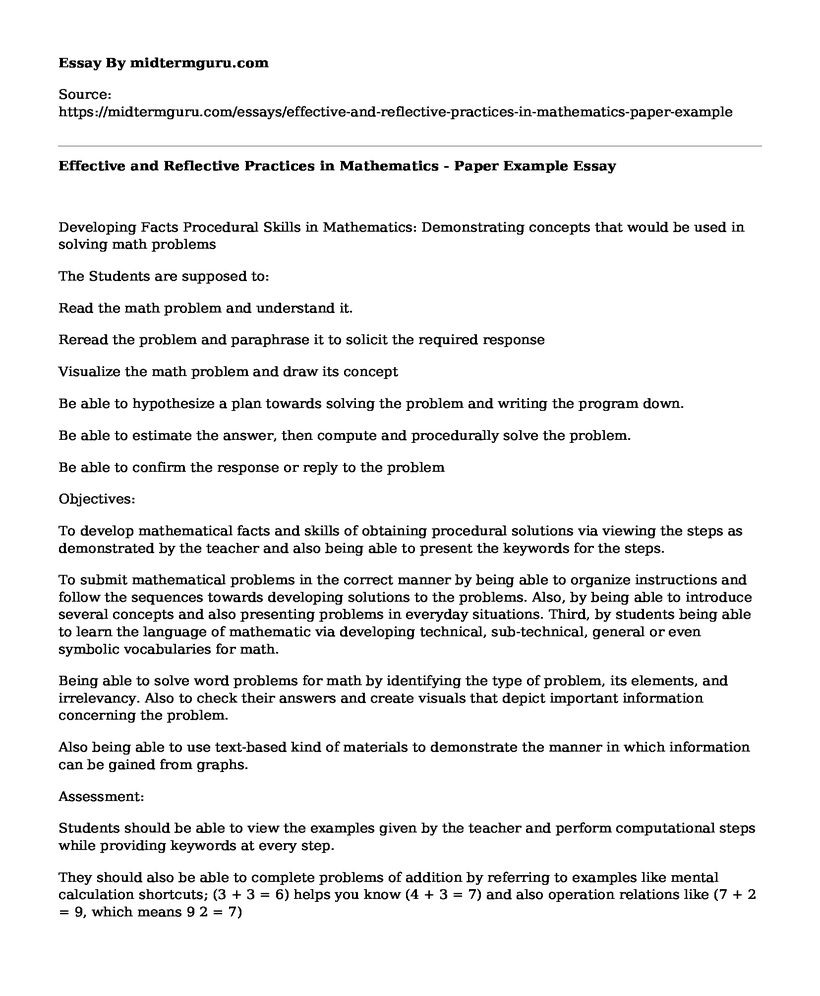# Effective and Reflective Practices in Mathematics - Paper Example

 Paper Type:  Presentation Pages:  3 Wordcount:  805 Words Date:  2021-06-17
Categories:

Developing Facts Procedural Skills in Mathematics: Demonstrating concepts that would be used in solving math problemsIs your time best spent reading someone else’s essay? Get a 100% original essay FROM A CERTIFIED WRITER!

The Students are supposed to:

Read the math problem and understand it.

Reread the problem and paraphrase it to solicit the required response

Visualize the math problem and draw its concept

Be able to hypothesize a plan towards solving the problem and writing the program down.

Be able to estimate the answer, then compute and procedurally solve the problem.

Be able to confirm the response or reply to the problem

Objectives:

To develop mathematical facts and skills of obtaining procedural solutions via viewing the steps as demonstrated by the teacher and also being able to present the keywords for the steps.

To submit mathematical problems in the correct manner by being able to organize instructions and follow the sequences towards developing solutions to the problems. Also, by being able to introduce several concepts and also presenting problems in everyday situations. Third, by students being able to learn the language of mathematic via developing technical, sub-technical, general or even symbolic vocabularies for math.

Being able to solve word problems for math by identifying the type of problem, its elements, and irrelevancy. Also to check their answers and create visuals that depict important information concerning the problem.

Also being able to use text-based kind of materials to demonstrate the manner in which information can be gained from graphs.

Assessment:

Students should be able to view the examples given by the teacher and perform computational steps while providing keywords at every step.

They should also be able to complete problems of addition by referring to examples like mental calculation shortcuts; (3 + 3 = 6) helps you know (4 + 3 = 7) and also operation relations like (7 + 2 = 9, which means 9 2 = 7)

Also be able to use calculators that are geared towards developing literacy in mathematics and solving problems. The calculators should also be able to help students crosscheck facts in computation and thus promoting dependency within themselves.

With the calculators, students are also expected to have the speed of solving math problems.

Resources: Spencer J. Salend. (2008). Creating Inclusive Classrooms: Effective and Reflective Practices (8th Edition).

Developed by: Marya Grande, Canisius College

Instructional Procedures

Introduction:

The learners should be able to tell the teacher if they have ever had terrible moments where they are faced with learning new math concepts. How do they go about tackling the tough math problems? Then the teacher should introduce a new concept towards solving particular math concepts. The students should be made to understand that the particular ideas that are presented are geared towards learning math issues and how to address the problems in mathematics.

The body of Lesson:

Step 1. Activate the background knowledge:

The first thing to do is to have all students pull out their math textbooks and then rehearse on how to read the digital text and also how to use numbering systems.

Step 2. Discussing the strategy for learning math

As an instructor, I would ensure that I fast go through the steps before reading them to the students and allowing them to write down the concepts. The strategies would include:

Testing the students' high-stakes

Testing the students accommodative level

Studying the skills as developed by the students

Creating accessible tests that are also valid

Showing the students alternative to working on their own

Step 3. Use of the strategies:

As a teacher, I would take my time to ensure that the details of the steps are thoroughly discussed and understood.

Step 4. Strategy memorization:

The students and I will take notes, use semantic webs, anticipation guides, acronyms, and acrostics to memorize the concepts used to solve the math problems.

Step 5. Support the utilization of the strategies:

At this point, other strategies and ways of solving and memorizing math solving concepts are discussed. They include:

Examining the exit tickets

This includes shooting a question and soliciting answers that provide contents that would possibly be used in future lessons.

Learning Logs

Students are given the opportunity to write comments regarding whatever they have learned, the manner in which they have heard it, whatever they never understood, what makes them confused and why, and also what sort of help do they still need.

Self Evaluation

Self-evaluation can provide information concerning the perceptions of students including their educational challenges, whatever the progress they make in learning math materials, and methods of task completing.

Conclusion:

Before the lesson is completed, I will have to question all the students concerning the procedures they underwent in the identification and learning of new concepts of solving math problems. My aim for doing so is to ensure that they can do perform the same math again all by themselves in the future. As the instructor, I will make sure that the steps are written down as they copy them directly from the whiteboard. I will also provide the necessary clarifications. Possible ways that would be used to solve math problems will be discussed both in the group and individual levels.

Effective and Reflective Practices in Mathematics - Paper Example. (2021, Jun 17). Retrieved from https://midtermguru.com/essays/effective-and-reflective-practices-in-mathematics-paper-exampleFree essays can be submitted by anyone,

so we do not vouch for their quality

Want a quality guarantee?
Order from one of our vetted writers instead

If you are the original author of this essay and no longer wish to have it published on the midtermguru.com website, please click below to request its removal:

Liked this essay sample but need an original one?

Hire a professional with VAST experience!